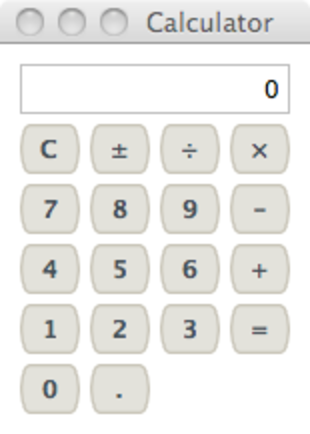# Re: Factor

Factor: the language, the theory, and the practice.

## Calculator with GUI

Monday, August 23, 2010

Update: Kyle Cordes has made some nice refactoring to avoid the “code smell” of passing global variables around while building the gadgets.

I started playing around with the Factor GUI framework recently. The documentation is very detailed, but sometimes it is nice to have simple examples to learn from.

I thought it would be fun to build a simple calculator application. A teaser of what it will look like when we are done:First, some imports and a namespace.

``````USING: accessors colors.constants combinators.smart kernel fry
math math.parser models namespaces sequences ui ui.gadgets

FROM: models => change-model ;

IN: calc-ui
``````

Note: we have to specifically import `change-model` from the `models` vocabulary, since it might conflict with an `accessor`.

Factor user interface elements are called gadgets. Many of them support being dynamically updated by being connected to models. Each model maintains a list of connections that should be updated when the value being held by the model changes.

### The Model

Our `calculator` model is based on the notion that we have two numbers (`x` and `y`) and an operator that can be applied to produce a new value.

``````TUPLE: calculator < model x y op valid ;

: <calculator> ( -- model )
"0" calculator new-model 0 >>x ;
``````

If we want to reset the model (such as when we press the “clear” button):

``````: reset ( model -- )
0 >>x f >>y f >>op f >>valid "0" swap set-model ;
``````

We’re storing all values as floating-point numbers, but (for display purposes) we’ll show integers when possible:

``````: display ( n -- str )
>float number>string dup ".0" tail? [
] when ;
``````

Each of `x` and `y` can be set based on the `value`, and the `op` is specified as a quotation:

``````: set-x ( model -- model )
dup value>> string>number >>x ;

: set-y ( model -- model )
dup value>> string>number >>y ;

: set-op ( model quot: ( x y -- z ) -- )
>>op set-x f >>y f >>valid drop ;
``````

Pushing the “=” button triggers the calculation:

``````: (solve) ( model -- )
dup [ x>> ] [ y>> ] [ op>> ] tri call( x y -- z )
[ >>x ] keep display swap set-model ;

: solve ( model -- )
dup op>> [ dup y>> [ set-y ] unless (solve) ] [ drop ] if ;
``````

We support negating the number:

``````: negate ( model -- )
dup valid>> [
[ [ 1 tail ] change-model ]
[ [ "-" prepend ] change-model ] if
] [ drop ] if ;
``````

And pushing the “.” button (to add a decimal), or a number (to add a digit):

``````: decimal ( model -- )
dup valid>
[ [ dup "." subseq? [ "." append ] unless ] change-model ]
[ t >>valid "0." swap set-model ] if ;

: digit ( n model -- )
dup valid>
[ swap [ append ] curry change-model ]
[ t >>valid set-model ] if ;
``````

That pretty much rounds out the basic features of the model.

### The GUI

For convenience, I store the calculator model in a global symbol:

``````SYMBOL: calc
<calculator> calc set-global
``````

I can use that to create buttons for each type (using short names and unicode characters to make the code a bit prettier):

``````: [C] ( -- button )
"C" calc get-global '[ drop _ reset ] <border-button> ;

: [±] ( -- button )
"±" calc get-global '[ drop _ negate ] <border-button> ;

: [+] ( -- button )
"+" calc get-global '[ drop _ [ + ] set-op ] <border-button> ;

: [-] ( -- button )
"-" calc get-global '[ drop _ [ - ] set-op ] <border-button> ;

: [×] ( -- button )
"×" calc get-global '[ drop _ [ * ] set-op ] <border-button> ;

: [÷] ( -- button )
"÷" calc get-global '[ drop _ [ / ] set-op ] <border-button> ;

: [=] ( -- button )
"=" calc get-global '[ drop _ solve ] <border-button> ;

: [.] ( -- button )
"." calc get-global '[ drop _ decimal ] <border-button> ;

: [#] ( n -- button )
dup calc get-global '[ drop _ _ digit ] <border-button> ;

: [_] ( -- label )
"" <label> ;
``````

We will create a label that is updated when the model changes.

``````: <display> ( -- label )
calc get-global <label-control> { 5 5 } <border>
{ 1 1/2 } >>align
COLOR: gray <solid> >>boundary ;
``````

And, finally, creating the GUI (using vertical and horizontal track layouts):

``````: <col> ( quot -- track )
vertical <track> 1 >>fill { 5 5 } >>gap
swap output>array [ 1 track-add ] each ; inline

: <row> ( quot -- track )
horizontal <track> 1 >>fill { 5 5 } >>gap
swap output>array [ 1 track-add ] each ; inline

: calc-ui ( -- )
[
<display>
[     [C]     [±]     [÷]    [×] ] <row>
[ "7" [#] "8" [#] "9" [#]    [-] ] <row>
[ "4" [#] "5" [#] "6" [#]    [+] ] <row>
[ "1" [#] "2" [#] "3" [#]    [=] ] <row>
[ "0" [#]     [.]     [_]    [_] ] <row>
] <col> { 10 10 } <border> "Calculator" open-window ;

MAIN: calc-ui
``````

Then, running the calculator application:

``````IN: scratchpad "calc-ui" run
``````

The code for this is on my GitHub.